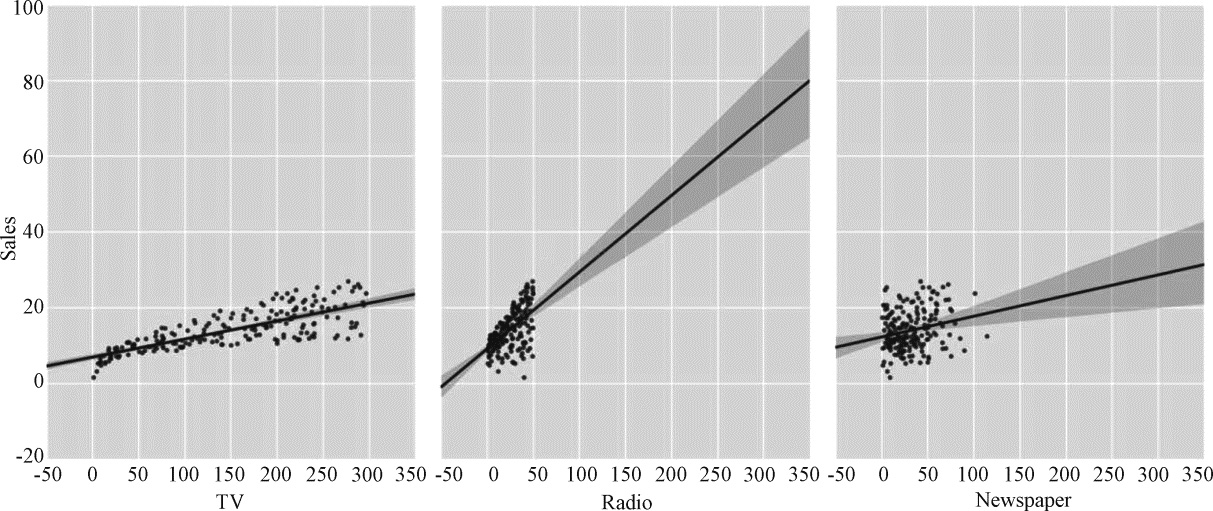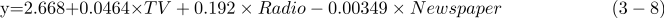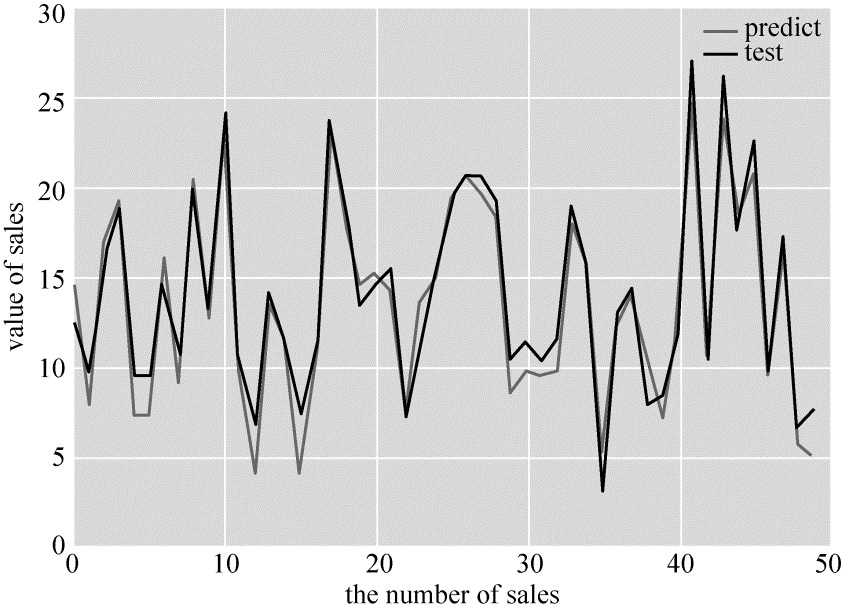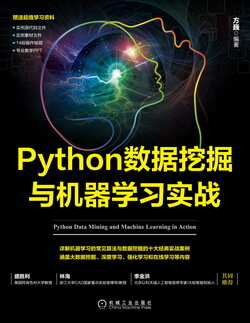# Python数据挖掘与机器学习实战（四）：用 Python 实现多元线性回归

2020 年 2 月 01 日## 3.4　用 Python 实现多元线性回归

Sales=β0+β1TV+β2Radio+β3Newspaper(37)$Sales=\beta_0+\beta_1TV+\beta_2Radio+\beta_3Newspaper\quad\quad\quad\quad\quad\quad(3-7)$

### 3.4.1　使用 pandas 读取数据

pandas 是一个用于数据探索、数据分析和数据处理的 Python 库。

import pandas as pd#获取数据data = pd.read_csv('/home/lulei/Advertising.csv')# 显示前 5 项数据data.head()

TV     Radio     Newspaper    Sales0      230.1      37.8       69.2       22.11      44.5       39.3       45.1       10.42      17.2       45.9       69.3       9.33      151.5      41.3       58.5       18.54      180.8      10.8       58.4       12.9

pandas 的两个主要数据结构是 Series 和 DataFrame；Series 类似于一维数组，它由一组数据及一组与之相关的数据标签（即索引）组成；DataFrame 是一个表格型的数据结构，它含有一组有序的列，每列可以是不同的值类型。DataFrame 既有行索引也有列索引，它可以被看做由 Series 组成的字典。

# 显示最后 5 项数据data.tail()

        TV     Radio    Newspaper    Sales195    38.2     3.7       13.8         7.6196    94.2     4.9       8.1          9.7197    177.0    9.3       6.4          12.8198    283.6    42.0      66.2         25.5199    232.1    8.6       8.7          13.4

data.shape

（200,4）

### 3.4.2　分析数据

TV：在电视上投资的广告费用（以千万元为单位）；

Newspaper：用于报纸媒体的广告费用；

Sales：对应产品的销量。

import seaborn as snsimport matplotlib.pyplot as plt# 使用散点图可视化特征与响应之间的关系sns.pairplot(data, x_vars=['TV','Radio','Newspaper'], y_vars='Sales',     size=7, aspect=0.8) plt.show()                                  #注意必须加上这一句, 否则无法显示#这里选择 TV?Radio?Newspaper 作为特征,Sales 作为观测值

seaborn 的 pairplot 函数绘制 X 的每一维度和对应 Y 的散点图。通过设置 size 和 aspect 参数来调节显示的大小和比例。通过加入一个参数 kind=‘reg’，seaborn 可以添加一条最佳拟合直线和 95% 的置信带。

sns.pairplot(data, x_vars=['TV','Radio','Newspaper'], y_vars='Sales',    size=7, aspect=0.8, kind='reg')plt.show()### 3.4.3　线性回归模型

• 优点：快速；没有调节参数；可轻易解释；可理解。
• 缺点：相比其他复杂一些的模型，其预测准确率不高，因为它假设特征和响应之间存在确定的线性关系，这种假设对于非线性的关系，线性回归模型显然不能很好地进行数据建模。

1．使用 pandas 构建 X（特征向量）和 y（标签列）

scikit-learn 要求 $\boldsymbol{X}$ 是一个特征矩阵，$\boldsymbol{y}$ 是一个 NumPy 向量。pandas 构建在 NumPy 之上。因此，$\boldsymbol{X}$ 可以是 pandas 的 DataFrame，$\boldsymbol{y}$ 可以是 pandas 的 Series，scikit-learn 可以理解这种结构。

#创建特征列表feature_cols = ['TV', 'Radio', 'Newspaper']#使用列表选择原始 DataFrame 的子集X = data[feature_cols]X = data[['TV', 'Radio', 'Newspaper']]# 输出前 5 项数据print (X.head())

print (type(X))print (X.shape)

      TV      Radio    Newspaper0    230.1     37.8       69.21    44.5      39.3       45.12    17.2      45.9       69.33    151.5     41.3       58.54    180.8     10.8       58.4<class 'pandas.core.frame.DataFrame'>(200, 3)

#从 DataFrame 中选择一个 Seriesy = data['Sales']y = data.Sales#输出前 5 项数据print (y.head())

0    22.11    10.42     9.33    18.54    12.9Name: Sales

2．构建训练集与测试集

<pre name="code" class="python"><span style="font-size:14px;">##构造训练    集和测试集from sklearn.cross_validation import train_test_split 	#这里是引用交叉验证X_train,X_test, y_train, y_test = train_test_split(X, y, random_state=1)# 75% 用于训练,25% 用于测试print (X_train.shape)print (y_train.shape)print (X_test.shape)print (y_test.shape)

(150,3)(150,)(50,3)(50,)

3．sklearn 的线性回归

from sklearn.linear_model import LinearRegressionlinreg = LinearRegression()model=linreg.fit(X_train, y_train)      				#线性回归print (model)print (linreg.intercept_)       		#输出结果print (linreg.coef_)

LinearRegression(copy_X=True, fit_intercept=True, normalize=False)2.66816623043[ 0.04641001  0.19272538 -0.00349015]

# 将特征名称与系数对应zip(feature_cols, linreg.coef_)

[('TV', 0.046410010869663267), ('Radio', 0.19272538367491721), ('Newspaper', -0.0034901506098328305)]4．预测

y_pred = linreg.predict(X_test)print (y_pred)print (type(y_pred))

[ 14.58678373   7.92397999   16.9497993   19.35791038  7.36360284  7.35359269   16.08342325  9.16533046   20.35507374  12.63160058  22.83356472  9.66291461   4.18055603   13.70368584  11.4533557  4.16940565   10.31271413  23.06786868  17.80464565  14.53070132  15.19656684  14.22969609  7.54691167   13.47210324  15.00625898  19.28532444  20.7319878   19.70408833  18.21640853  8.50112687  9.8493781    9.51425763   9.73270043   18.13782015  15.41731544  5.07416787   12.20575251  14.05507493  10.6699926   7.16006245  11.80728836  24.79748121  10.40809168  24.05228404  18.44737314  20.80572631  9.45424805   17.00481708  5.78634105   5.10594849]<type 'numpy.ndarray'>

5．评价测度

• 平均绝对误差（Mean Absolute Error，MAE）；
• 均方误差（Mean Squared Error，MSE）；
• 均方根误差（Root Mean Squared Error，RMSE）。

#计算 Sales 预测的 RMSEprint (type(y_pred),type(y_test))print (len(y_pred),len(y_test))print (y_pred.shape,y_test.shape)from sklearn import metricsimport numpy as npsum_mean=0for i in range(len(y_pred)):    sum_mean+=(y_pred[i]-y_test.values[i])**2sum_erro=np.sqrt(sum_mean/50)# 计算 RMSE 的大小print ("RMSE by hand:",sum_erro)

<type 'numpy.ndarray'><class 'pandas.core.series.Series'>50 50(50,) (50,)RMSE by hand: 1.42998147691

import matplotlib.pyplot as pltplt.figure()plt.plot(range(len(y_pred)),y_pred,'b',label="predict")plt.plot(range(len(y_pred)),y_test,'r',label="test")plt.legend(loc="upper right")                             #显示图中的标签plt.xlabel("the number of sales")                         #横坐标轴plt.ylabel('value of sales')                              #纵坐标轴plt.show()#显示结果Python 数据挖掘与机器学习实战（一）：Python 语言优势及开发工具

Python 数据挖掘与机器学习实战（二）：Python 语言简介

Python 数据挖掘与机器学习实战（三）：网络爬虫原理与设计实现

Python 数据挖掘与机器学习实战（四）：用 Python 实现多元线性回归

Python 数据挖掘与机器学习实战（五）：基于线性回归的股票预测

2020 年 2 月 01 日 16:20 190

## 评论

• ###### 27 丨 K-Means（下）：如何使用 K-Means 对图像进行分割？

今天我们继续用K-Means进行聚类的实战。聚类的一个常用场景就是对图像进行分割。

2019 年 2 月 13 日

• ###### 如何对数据进行分类和预测？

分类也是大数据常见的应用场景之一，通过对历史数据规律的统计，将大量数据进行分类然后发现数据之间的关系。

2019 年 1 月 22 日

• ###### 41 丨数据挖掘实战（3）：如何对比特币走势进行预测？

今天我带你用数据挖掘对比特币的走势进行预测和分析，采用哪种方法比较好呢？

2019 年 3 月 18 日

• ###### 18 丨决策树（中）：CART，一棵是回归树，另一棵是分类树

CART决策树既可以做回归树，也可以做分类树。

2019 年 1 月 23 日

Java 面试

#### 我精心整理的 136 页 Excel 数据透视表 PDF 文件！【附获取方式】